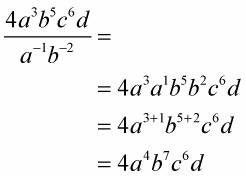##### Basic Math & Pre-Algebra For Dummies Book + Workbook Bundle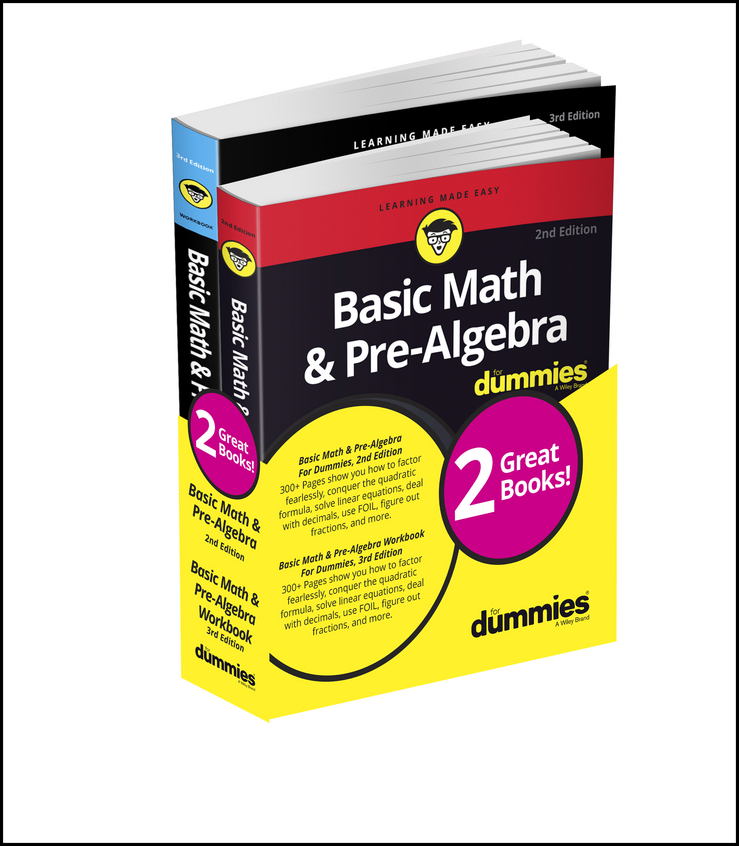Negative exponents are a way of writing powers of fractions or decimals without using a fraction or decimal. You use negative exponents as a way to combine expressions with the same base, whether the different factors are in the numerator or denominator. It's a way to change division problems into multiplication problems.

Example: Instead of writing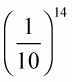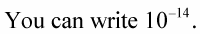A reciprocal of a number is the multiplicative inverse of the number. The product of a number and its reciprocal is equal to 1.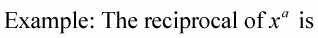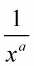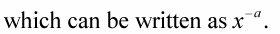The variable x is any real number except 0, and the exponent a is any real number. And, going to the negative side,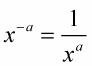The following examples show you how to change from positive to negative exponents, and vice versa.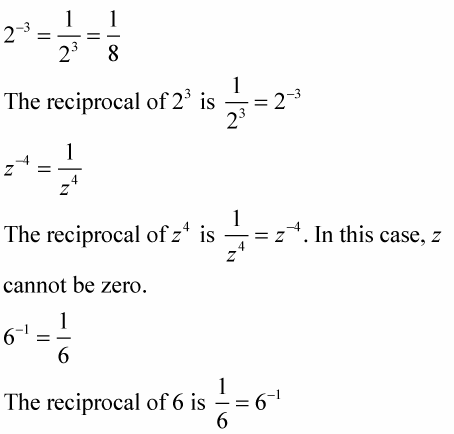If you start out with a negative exponent in the denominator, then the negative exponent in the denominator comes up to the numerator with a change in the sign to a positive exponent. For example,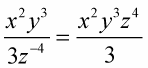Then you get,